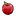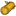## FANDOM

185 个页面

### 概要

• 一但你摧毀敵人城堡就會獲得獎勵寶箱，其內容取決於敵人的實力等級(Power Level)。
• 獲勝的程度(星星數、摧毀建築百分比)和獎勵寶箱內容並無關係
• 將可在時代三開始獲得文明旗幟與黃金。
• 將可在時代四開始獲得石頭。
• 即使沒有研發獎勵寶箱加成，也可以獲得白金寶箱。

### 公式

• 假設N為敵人的實力等級。 (1 ≤ N ≤ 30).
• 在遊戲一開始時，實力等級為1。如果你摧毀一個實力等級為一的敵人，你將會獲得85和 85• 另一範例：若你摧毀一位時代八的敵人，且其實力等級為25，你的獎勵寶箱加成也達到最大研究等級，則你將會在獎勵寶箱中獲得1525和/或 1525和/或 765和/或 26和/或 29.
• 白金寶箱獎賞是一般獎勵寶箱的三倍！！

#### 寶箱= 25 + N * 60= 25 + N * 60= 15 + N * 30= N + 1= N + N * 獎勵寶箱加成

#### 白金寶箱= (25 + N * 60) * 3= (25 + N * 60) * 3= (15 + N * 30) * 3= (N+1) * 3= (N + N * 獎勵寶箱加成) * 3